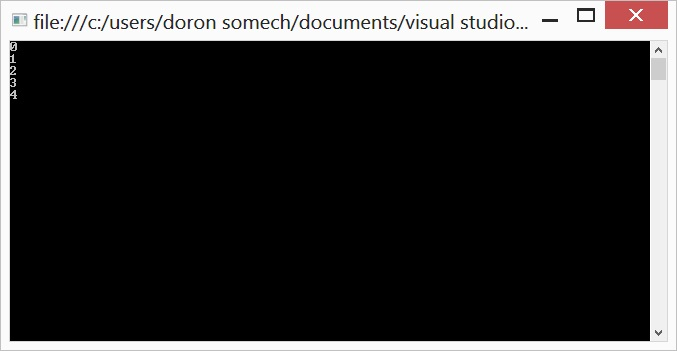# Array Length in C#

As you know in C# you can query the length of the array with Length property, but it’s not so obvious, in c++ for example you allocate the array to the size you want but the length is unknown after the allocation.

My question is, how in C# the language can tell you the length of the array, or to ask this question in another way, where does C# save the array length, my first guess was that the first item in an array is the array length, I decide to go and search for it. First I tried to search in the first item:

``````  static void Main(string[] args)
{
int[] array = new int;

array = 0;
array = 1;
array = 2;
array = 3;
array = 4;

var handle = GCHandle.Alloc(array, GCHandleType.Pinned);

IntPtr address = handle.AddrOfPinnedObject();

int value = Marshal.ReadInt32(address);
Console.WriteLine(value);

address += 4;
value = Marshal.ReadInt32(address);
Console.WriteLine(value);

address += 4;
value = Marshal.ReadInt32(address);
Console.WriteLine(value);

address += 4;
value = Marshal.ReadInt32(address);
Console.WriteLine(value);

address += 4;
value = Marshal.ReadInt32(address);
Console.WriteLine(value);

handle.Free();

Console.ReadLine();
}
``````

And the output:So you can see my guess was wrong, the first item is actually the first item.

Then I tried the 7 item (last + 1), which make no sense because it’s has to be the first otherwise the language won’t know where to search for it.

Finally I tried to search before the first item which actually prove right:

``````  static void Main(string[] args)
{
int[] array = new int;

array = 0;
array = 1;
array = 2;
array = 3;
array = 4;

var handle = GCHandle.Alloc(array, GCHandleType.Pinned);

IntPtr address = handle.AddrOfPinnedObject();

int value = Marshal.ReadInt32(address-4);
Console.WriteLine(value);

value = Marshal.ReadInt32(address);
Console.WriteLine(value);

address += 4;
value = Marshal.ReadInt32(address);
Console.WriteLine(value);

address += 4;
value = Marshal.ReadInt32(address);
Console.WriteLine(value);

address += 4;
value = Marshal.ReadInt32(address);
Console.WriteLine(value);

address += 4;
value = Marshal.ReadInt32(address);
Console.WriteLine(value);

handle.Free();

Console.ReadLine();
}
``````

To summarize C# save the length of the array one item before the first item of the array (or 4 bytes before the beginning of the array).
Which actually make the size of the array num_of_items * size_of_item + 4 (which is size of integer holding the array size).